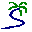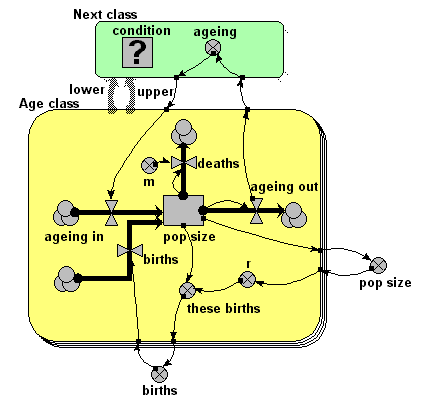# Model entry

Age-class model of population dynamics, using a multiple-instance submodel and an association submod - Model catalogue - Simulistics.com

 Search Simulistics Model catalogue Listed by keyword Listed by ID Listed by title Listed by date added

# Age-class model of population dynamics, using a multiple-instance submodel and an association submod

Model : ageclass6
Simile version : 3.1+
Keywords : Age-class modelling ; Population dynamics ; Association submodel ;

## Description

This models a population of animals in terms of four age-classes. For each age-class, we use a compartment (state variable) to represent the number of individuals in that class.

This version uses a four-instance submodel to represent the four age-classes. The ageing flow (from one class to the next) thus has to be handled by transferring the value for the outflow from each class to be assigned to the inflow for the next class. In this model, this is done by using an association submodel to represent the fact that each age class is 'associated' with the next one (by virtue of the fact that the next class receives the animals that have aged out of the previous class). The value for the number of animals leaving progressing from one class to the next is then passed through this submodel.

## Files

### Model file

Click on the icon to download the model file. (You will need Simile to examine and run the model. A free evaluation version is available from the products page.)

Some browsers may attempt to display the model file, rather than open it in Simile; in this case, use the browser back button to return to this page, and use the context menu (invoked by right-clicking on the link) to save the target file to disk.ageclass6.sml

## Diagram## Equations

```Equations in Desktop

births = sum([these_births])
pop size = sum([pop_size])

Equations in Age class

pop size:  initial value = element([2,0,0,0],index(1))

ageing in = sum({ageing_next})
Where: {ageing_next}=../Next class/ageing (to Age class in next)

ageing out = element([1,0.2,0.1,0],index(1))*pop_size

births = if index(1)==1 then births else 0

deaths = m*pop_size

class = index(1)

m = element([0.05,0.01,0.01,0.05],index(1))

r = element([0,0.05,0.2,0.1],index(1))*(1-total_pop_size/100)

these births = r*pop_size

Equations in Next class

condition = class_next==class_this+1
Where:
class_this=../Age class/class (from Age class in this)
class_next=../Age class/class (from Age class in next)

ageing = ageing_out_this
Where: ageing_out_this=../Age class/ageing out (from Age class in this)
```

## Results

||